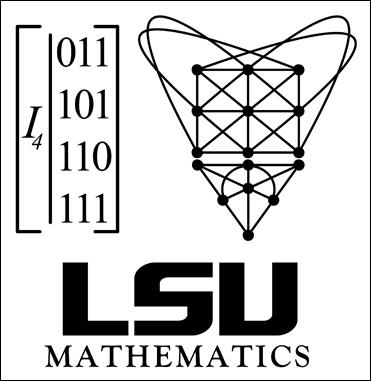High School Mathematics ContestContest Home > Contest Logo -> 2011 Contest Logo

## 2011 Contest LogoWe owe the T-shirt design and our 2011 contest logo to Dennis Hall and Jesse Taylor, graduate students in combinatorics of LSU Math Department.

Projective and Affine Geometries are geometries that are different from the usual Euclidean Geometry students learn in high school. A major difference between these is that while in Euclidean Geometry, you are able to find the distance between two points, Projective and Affine Geometries have no sense of distance. Further, Projective and Affine Geometries have no measure of angles between lines, and don't even have to be infinite! However, Affine Planes do share with Euclidean Planes the fact that any two lines either intersect at a point or are parallel. Projective Planes are a bit more restricted: any two lines will always intersect at a point.

The top portion of the heart is an affine plane with 9 points and 12 lines. The bottom portion is a projective plane with 7 points and 7 lines. There are several spots on each of these that look like lines cross, but they do not. The only intersections are at the bold points. On the affine plane, try finding two parallel lines. On the projective plane, check that all the lines intersect at exactly one point.

This drawing is only one way to display the information in a projective geometry. Often, it is either impossible to make a drawing or it is more convenient to have the information presented in another manner. The "I" in the drawing is a matrix representation of the 7 element projective geometry drawn in the bottom portion of the heart. To see this, number each of the columns of the matrix 1 through 7. Consider all new rows that can be obtained by adding rows together where two rows are added by adding corresponding entries. By doing these additions modulo two (so 1+1=0), we can find exactly 16 different rows by successive additions. Of these 16 rows, there are 7 with exactly three ones. These represent the 7 lines. Try labeling the points in the drawing so that the 7 lines in the picture match up with the 7 rows that have exactly three nonzero entries.Contest organizer: Contest e-mail:   Contest web-page: Mark Davidson, phone: (225) 578-1581 contest@math.lsu.edu www.math.lsu.edu/~contest